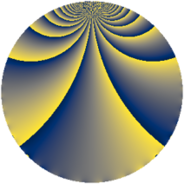# Properties

 Label 3630.2.uLevel $3630$ Weight $2$ Character orbit 3630.u Rep. character $\chi_{3630}(331,\cdot)$ Character field $\Q(\zeta_{11})$ Dimension $880$ Sturm bound $1584$

# Related objects

## Defining parameters

 Level: $$N$$ $$=$$ $$3630 = 2 \cdot 3 \cdot 5 \cdot 11^{2}$$ Weight: $$k$$ $$=$$ $$2$$ Character orbit: $$[\chi]$$ $$=$$ 3630.u (of order $$11$$ and degree $$10$$) Character conductor: $$\operatorname{cond}(\chi)$$ $$=$$ $$121$$ Character field: $$\Q(\zeta_{11})$$ Sturm bound: $$1584$$

## Dimensions

The following table gives the dimensions of various subspaces of $$M_{2}(3630, [\chi])$$.

Total New Old
Modular forms 8000 880 7120
Cusp forms 7840 880 6960
Eisenstein series 160 0 160

## Trace form

 $$880q - 88q^{4} + 880q^{9} + O(q^{10})$$ $$880q - 88q^{4} + 880q^{9} - 4q^{10} - 12q^{11} + 64q^{13} + 64q^{14} - 88q^{16} - 32q^{17} - 8q^{19} - 8q^{22} - 16q^{23} - 88q^{25} - 8q^{26} - 16q^{29} - 4q^{30} + 48q^{31} - 8q^{33} - 8q^{34} - 8q^{35} - 88q^{36} - 16q^{37} - 16q^{38} - 8q^{39} + 40q^{40} - 24q^{41} - 24q^{43} - 12q^{44} - 16q^{46} - 16q^{47} - 40q^{49} - 32q^{51} - 24q^{52} + 56q^{53} - 12q^{55} - 24q^{56} - 8q^{57} + 72q^{58} - 24q^{59} - 64q^{61} - 16q^{62} - 88q^{64} - 8q^{65} - 12q^{66} + 72q^{67} - 32q^{68} - 16q^{69} - 48q^{71} - 8q^{73} - 40q^{74} + 36q^{76} - 80q^{77} + 88q^{79} + 880q^{81} + 40q^{82} - 112q^{83} - 8q^{85} - 16q^{86} - 16q^{87} - 8q^{88} + 72q^{89} - 4q^{90} + 184q^{91} - 16q^{92} - 16q^{93} + 128q^{94} + 200q^{97} - 32q^{98} - 12q^{99} + O(q^{100})$$

## Decomposition of $$S_{2}^{\mathrm{new}}(3630, [\chi])$$ into newform subspaces

The newforms in this space have not yet been added to the LMFDB.

## Decomposition of $$S_{2}^{\mathrm{old}}(3630, [\chi])$$ into lower level spaces

$$S_{2}^{\mathrm{old}}(3630, [\chi]) \cong$$ $$S_{2}^{\mathrm{new}}(121, [\chi])$$$$^{\oplus 8}$$$$\oplus$$$$S_{2}^{\mathrm{new}}(242, [\chi])$$$$^{\oplus 4}$$$$\oplus$$$$S_{2}^{\mathrm{new}}(363, [\chi])$$$$^{\oplus 4}$$$$\oplus$$$$S_{2}^{\mathrm{new}}(605, [\chi])$$$$^{\oplus 4}$$$$\oplus$$$$S_{2}^{\mathrm{new}}(726, [\chi])$$$$^{\oplus 2}$$$$\oplus$$$$S_{2}^{\mathrm{new}}(1210, [\chi])$$$$^{\oplus 2}$$$$\oplus$$$$S_{2}^{\mathrm{new}}(1815, [\chi])$$$$^{\oplus 2}$$